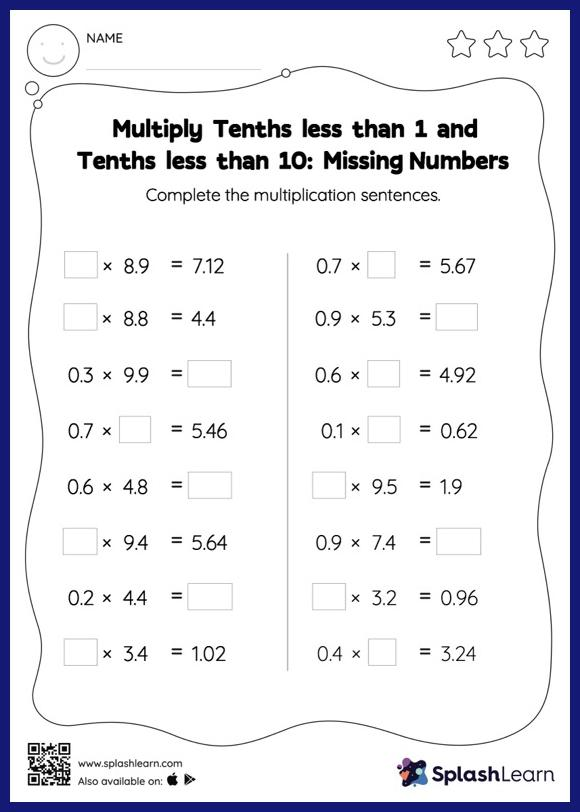# Multiply Tenths less than 1 and Tenths less than 10: Missing Numbers Worksheet

Home > Multiply Tenths less than 1 and Tenths less than 10: Missing NumbersMultiply tenths less than 1 and tenths less than 10 worksheet is a great way to help learners become fluent with the concept of multiplication. As the worksheet uses a horizontal format, it allows for more creativity when it comes to how the student solves the problem. In contrast, in the vertical format, students usually employ the standard method to solve.